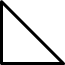Register for Maths, Science, English, Reasoning Olympiad Exams Register here | Book Free Demo for Live Olympiad Classes here | Check Olympiad Exam Dates here | Buy Practice Papers for IMO, IOM, HEO, IOEL etc here | Login here to participate in all India free mock test on every Saturday

# Geometry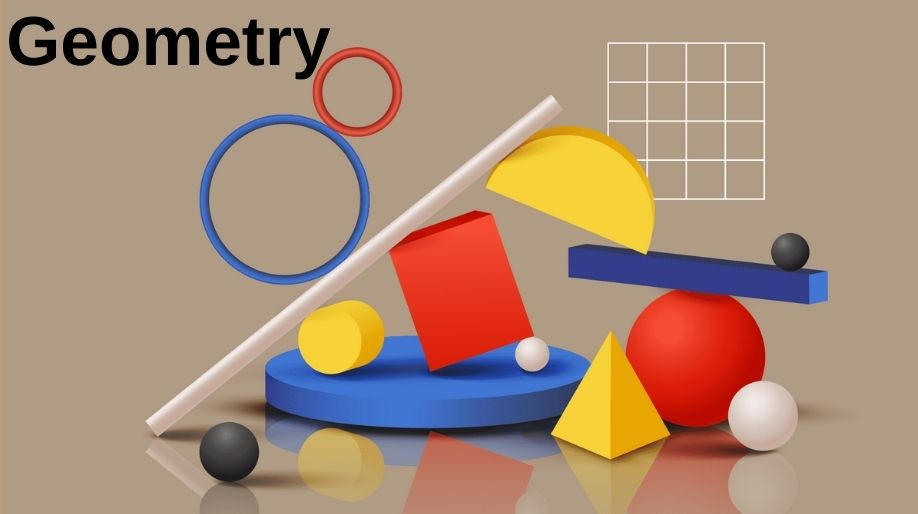When we look around we see objects of different shapes, sizes and there are different figures having similarities and differences also. But here a question arises; how do we find out which figure is that and how do we obtain that shape? Let’s learn about all these queries.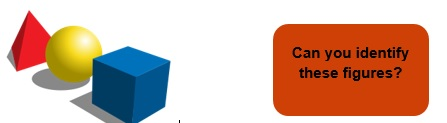Geometry is a branch of mathematics that deals with measurements and properties of different points, lines, shapes, angles, etc.

Let’s learn some basic shapes and their definitions:

Points: A point is any location on space having no length, breadth and height that is points are dimensionless.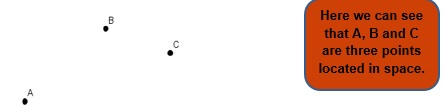Ray: A ray is a straight path extended to only a single direction and has no end points.

Line: A line is a straight path extended to both the directions and it also does not have any end points. It is one dimensional.

Line Segment: It is a part of line having two definite end points.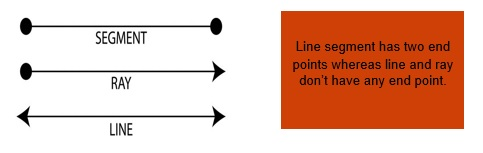Curved Line: It is as similar to a line but here it is not straight which means curved and like line it may have end points or not.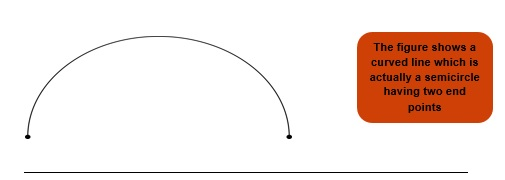Let’s learn about some basic shapes and where do we use them:

Circle

It is simple closed shape where each and every point is at a same distance from a fixed point which is called as Centre.

It has no sides, no vertices. It only has a radius which is the distance of any point on the circle from Centre.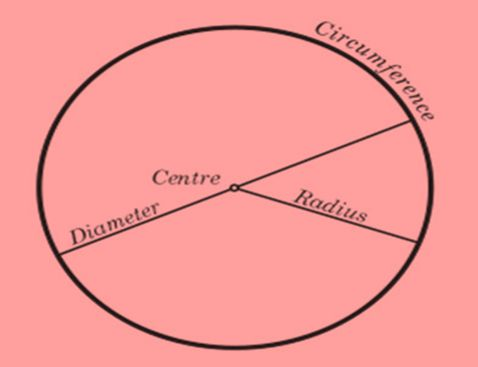• The boundary is known as circumference.

• The distance of any point on boundary from Centre is known as Radius(R) and twice of radius is called as Diameter (D).

Formula:

Diameter = 2 x R

Circumference = 2 x π x R

Area = π x R x R

Example 1: Find the circumference and area of a circle of radius 4 cm.

Solution: As we know that here R= 4 cm.

Circumference = 2 x π x R = 2 x π x 4 = 8 x 3.14 = 25.12 cm.

Area = π x R x R = 3.14 x 4 x 4 = 50.24 cm².

Example 2: Find the radius of the circle of diameter 24 cm.

Solution: As we know that diameter = 2R

Therefore R = D ÷ 2 = 24 ÷2 cm = 12 cm.

Angles

When two rays are drawn in different directions from a single point, the rays are said to be at an angle to each other.

• The point is called vertex.

• If the angle is measured in clockwise directions it is positive angle.

• If the angle is measured in anticlockwise direction it is said to be negative angle.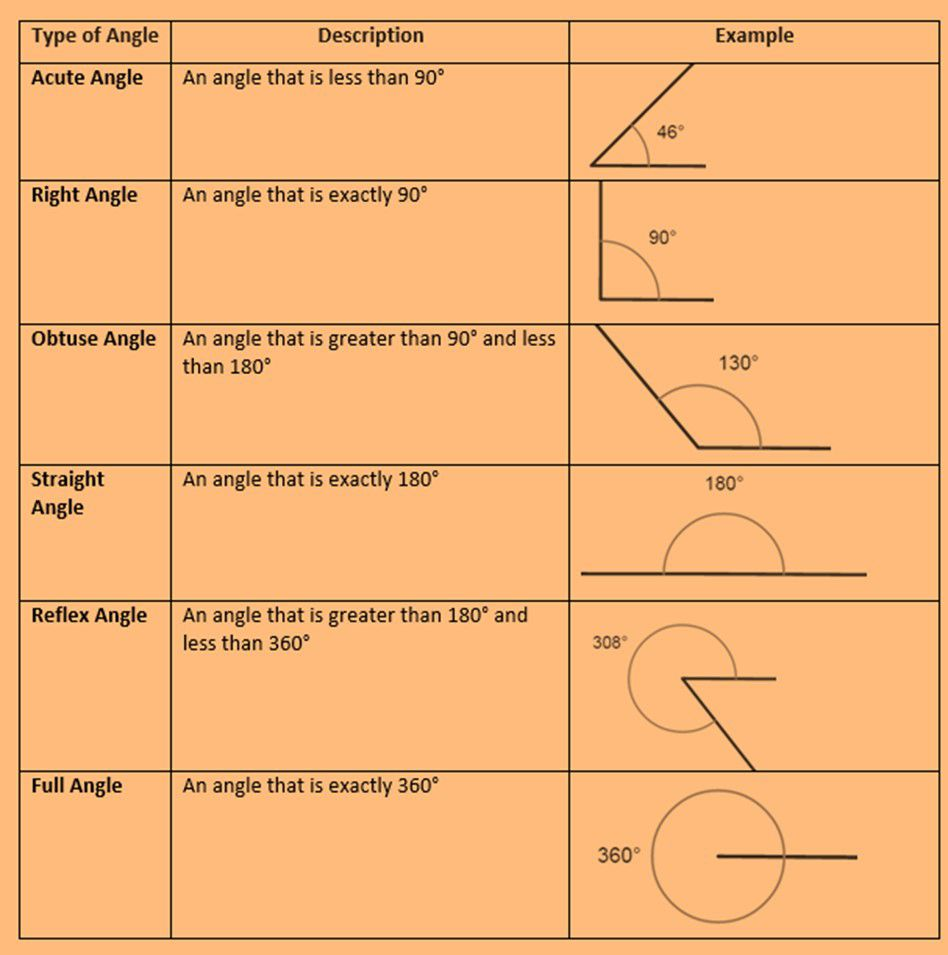Triangle

A closed figure containing three sides is called a triangle.

• The sum of all interior angles is 180°.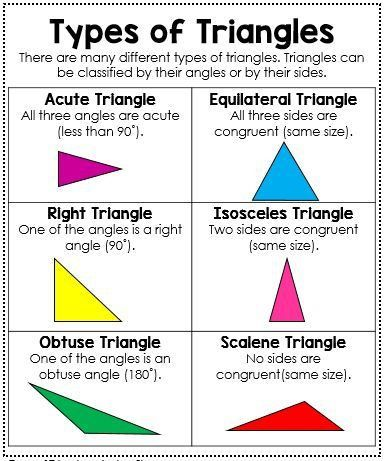A closed shape having four sides is known as a quadrilateral.

• The interior angles between the sides vary according to different shapes.
• Each point where the two sides meet is called as vertex.

Square and Rectangle are also quadrilaterals.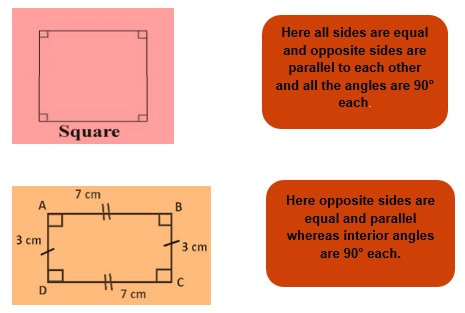Formulas:

Perimeter of Square = 4 x (length of side)

Area of Square = (length of side) x (length of side)

Perimeter of Rectangle = 2 x (Length + Breadth)

Example 3: Find the perimeter and area of square of side 3 cm.

Solution: Perimeter = 4 x (3 cm) = 12 cm

Area = 3 cm x 3 cm = 9 cm².

Example 4: Find the perimeter and area of rectangle of length 4 cm and breadth 2 cm.

Solution: Perimeter = 2 x (4 cm + 2 cm) = 12 cm.

Area = 4 cm x 2 cm = 8 cm².

Practice Questions

Q1) Find the area of the circle of diameter 100 cm.

Q2) Find the radius of the circle if the circumference is 62.8 cm.

Q3) Find the perimeter of the square if its side is 14 cm.

Q4) Find the area of a rectangle if its length is 6 cm and the perimeter is 12 cm.

Q5) State True or False:

1. In acute angle triangle each angle is 60°.
2. The sum of all the angles is 180° in a triangle.
3. In an isosceles triangle one angle is 90°.
4. If an angle is greater than 90° then it is acute.
5. If an angle is 90° then it is right angle.
6. A straight line has 360° angle.

Q6) Fill in the blanks:

1. The sum of all the angles of a triangle is
2. Each angle measures in an equilateral triangle.
3. Each angle is 90° in an acute angle triangle.

Recap

• Points are dimensionless.
• Ray and line don’t have any end points.
• Curved line can never be straight.
• In a circle Diameter = 2Radius.
• Area of circle =
• Circumference of circle = .
• Rectangle has only opposite sides equal whereas a square has all sides equal.

## Quiz for Geometry

 Q.1 Find a shape which has more than 5 sides. a)b)c)d)Q.2 Find a shape which has more than 3 sides. a)b)c)d)Q.3 Find a shape which has more than 4 sides. a)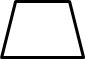b)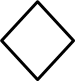c)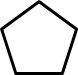d)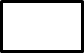Q.4 Find a shape which has 3 corners. a)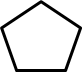b)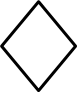c)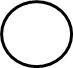d)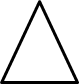Q.5 Find a shape which has more than 4 corners. a)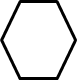b)c)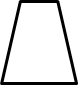d)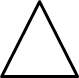Q.6 Find a shape which has 5 corners. a)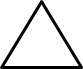b)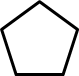c)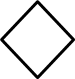d)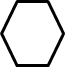Q.7 Find the odd one out. a)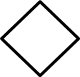b)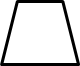c)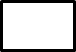d)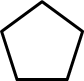Q.8 Find the odd one out. a)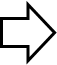b)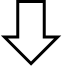c)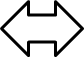d)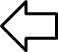Q.9 Find the odd one out. a)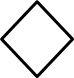b)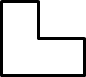c)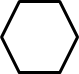d)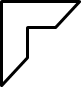Q.10 Which figure is similar to the given figure?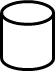a)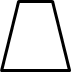b)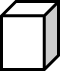c)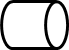d)